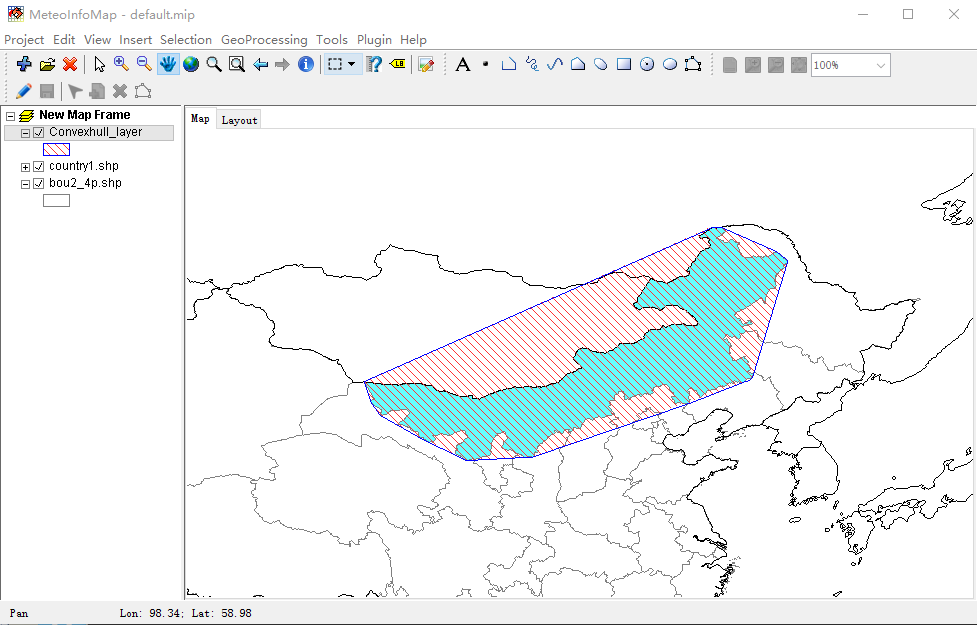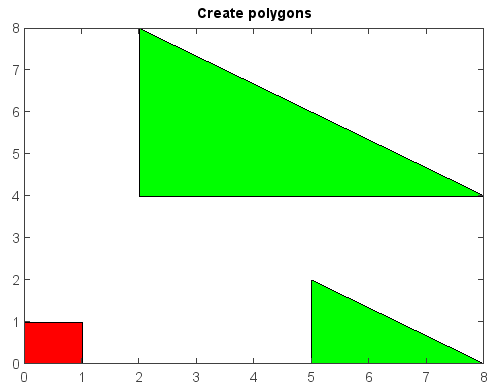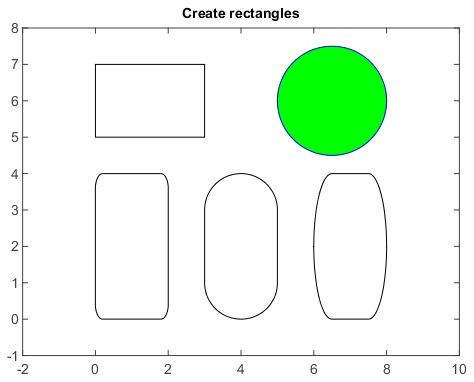# MeteoInfo 1.4.3 was released (2017-5-4)¶

• MeteoInfo GIS desktop application is renamed as MeteoInfoMap and associated commands are mimap.exe, mimap.sh and mimap_mac.sh.

• In MeteoInfoMap, Convexhull and Intersection functions were added under Geoprocessing menu.

Convexhull function example:• patch and rectangle functions were added in miplot module for creating polygons and rectangles.

Create polygons:

x = [0, 1, 1, 0]
y = [0, 0, 1, 1]
patch(x, y, facecolor='red')
x2 = [2, 2, 8, nan, 5, 5, 8]
y2 = [4, 8, 4, nan, 0, 2, 0]
patch(x2, y2, facecolor='green')
title('Create polygons')Create rectangles. curvature augument is used to draw rectangle with curved corners or circle.

axes(aspect='equal')
rectangle([0, 5, 3, 2])
rectangle([0, 0, 2, 4], curvature=0.2)
rectangle([3, 0, 2, 4], curvature=[1, 0.5])
rectangle([6, 0, 2, 4], curvature=[0.5, 1])
rectangle([5, 4.5, 3, 3], curvature=1, facecolor='g', edgecolor='b')
xlim(-2, 10)
ylim(-1, 8)
title('Create rectangles')